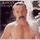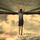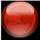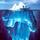## General Question# Why does any number raised to an exponent of zero equal 1?

Asked by cockswain (15271) September 5th, 2010

Just wondering. A while back I asked why division by zero was undefined and got some great answers. I’ve pondered this one and haven’t figured out the logic behind it.

Observing members: 0Composing members: 0I can’t remember the math proving it, but I sort of remember when I questioned it myself when doing A-levels maths that I realised that it equals the number being divided by itself, which is always 1.

Ok I just took a quick look back and here is my “proof” or reasoning.

2×2x2 = 2^3 = 8
2×2 = 2^2 = 4
2 = 2^1 = 2
2/2 = 2^0 = 1
2/2^2 (or 2/2 divided by 2 again) = 2^-1 = ½

Basically the powers are on a scale shown above, and ^0 is just between 1 and -1 which means that the number is divided by itself. This doesn’t work for 0 though because 0/0 = impossible or infinity depending who you listen to.

Also I am unsure whether this works for negative numbers, so please let me know as I do not have any proper calculator to do this with.

Ame_Evil (3046)“Great Answer” (3) Flag as…jackm (6205)“Great Answer” (4) Flag as…@jackm Great link. Here’s the key:

This fraction equals 1, because the numerator and the denominator are the same. If we apply the law of exponents, we get:
3^4
1 =—- = 3^(4–4) = 3^0
3^4
So 3^0 = 1.

cockswain (15271)“Great Answer” (0) Flag as…Another way of looking at it:
3^a = 3^(a+0)=3^a * 3^0,
so 3^a = 3^a * 3^0
Dividing both sides by 3^a gives 3^0=1

It is interesting to see how exponentiation converts rules for addition into rules for multiplying.
0 is called the additive identity because for any number x, x+0=x
x^0 is the mulitplicative identity 1

Similarly, -a is the additive inverse of a since a + (-a) = 0
x^ -a = 1/a, the multiplicative inverse of x^a

LostInParadise (28217)“Great Answer” (1) Flag as…Correction: x^-a = 1/x^a, which is the mutiplicative inverse of x^a

LostInParadise (28217)“Great Answer” (0) Flag as…Here’s a different way of looking at it:

The fundamental idea is that 1 is the multiplicative identity. You can always multiply a number by 1 without changing it. Factors of 1 are always present in every product, and may occur any number of times. That’s why they’re normally ignored when factoring.

Now consider an exponent to be the number of times the base number appears as a factor. If it appears zero times, then all that remains are factors of 1 whose product is 1.

gasman (11313)“Great Answer” (4) Flag as…@gasman I really like that thought process.

jackm (6205)“Great Answer” (1) Flag as…gasman (11313)“Great Answer” (1) Flag as…@gasman I second @jackm. That is a cool way to look at it. I’m taking calculus now and am trying to fully grasp how to manipulate logs and exponents all around right now. Tips like that a very helpful (as will be any others you think of regarding what I’m working on).

cockswain (15271)“Great Answer” (1) Flag as…or